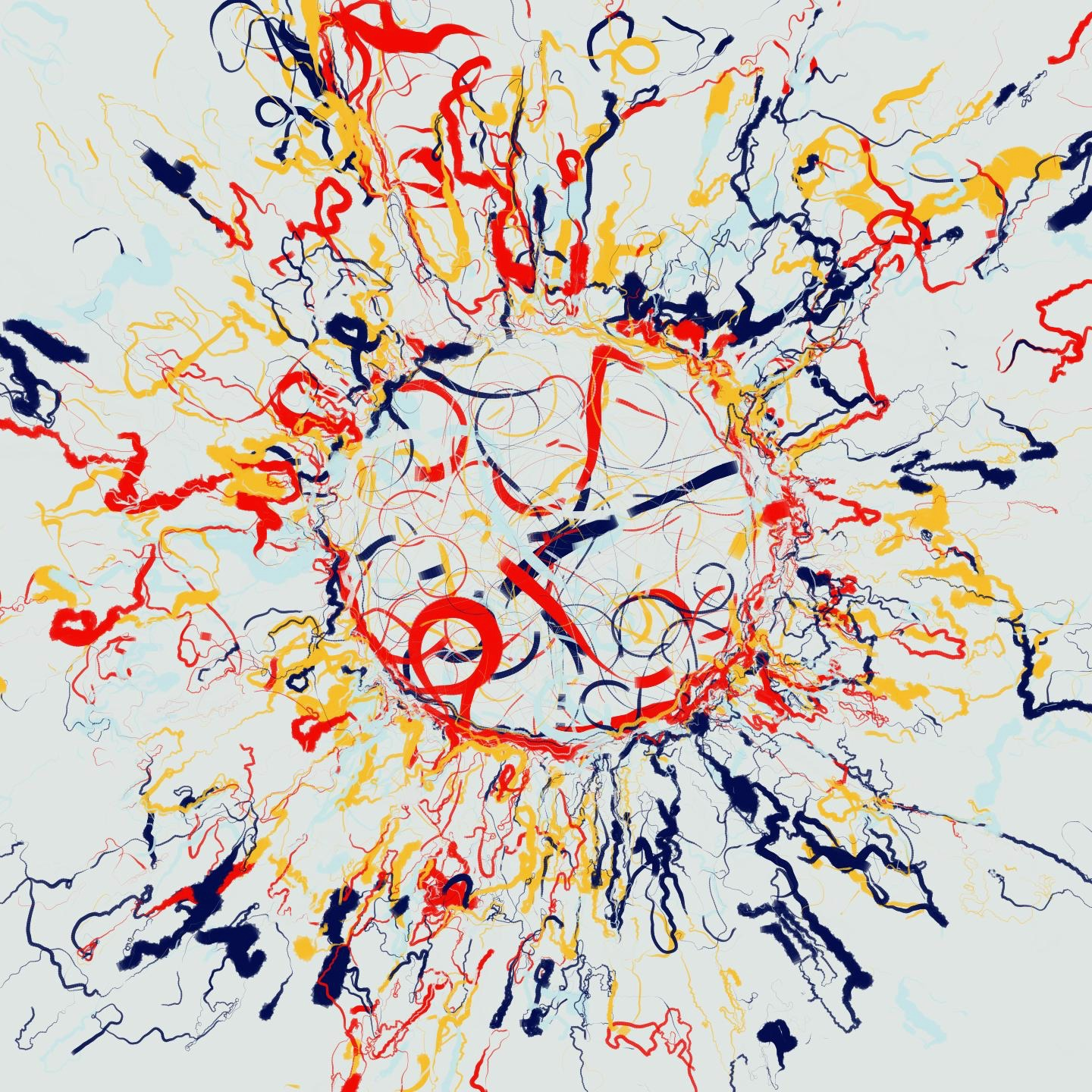# Distress propagation in complex networks: the case of non-linear DebtRank

Non-linear models of distress propagation in financial networks characterise key regimes where shocks are either amplified or suppressed.

PLoS ONE 1, 1 (2016)

M. Bardoscia, F. Caccioli, J. Perotti, G. Vivaldo, G. CaldarelliWe consider a dynamical model of distress propagation on complex networks, which we apply to the study of financial contagion in networks of banks connected to each other by direct exposures. The model that we consider is an extension of the DebtRank algorithm, recently introduced in the literature. The mechanics of distress propagation is very simple: When a bank suffers a loss, distress propagates to its creditors, who in turn suffer losses, and so on. The original DebtRank assumes that losses are propagated linearly between connected banks. Here we relax this assumption and introduce a one-parameter family of non-linear propagation functions. As a case study, we apply this algorithm to a data-set of 183 European banks, and we study how the stability of the system depends on the non-linearity parameter under different stress-test scenarios. We find that the system is characterized by a transition between a regime where small shocks can be amplified and a regime where shocks do not propagate, and that the overall stability of the system increases between 2008 and 2013.